# Mathematica绘制动态蔓叶线

## 三、怎么绘制动态蔓叶线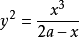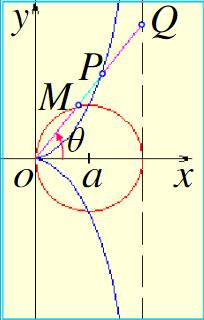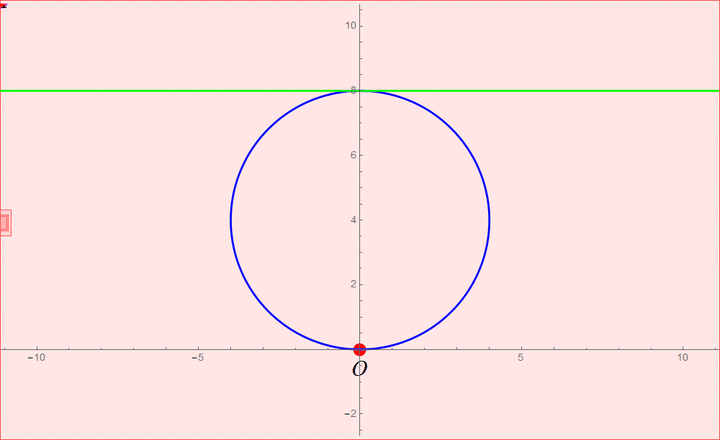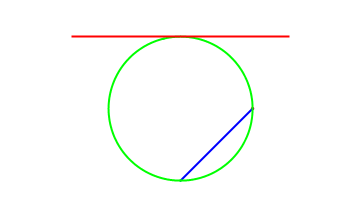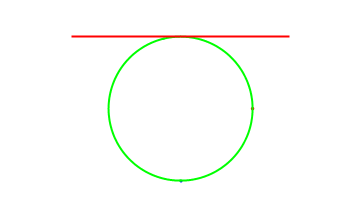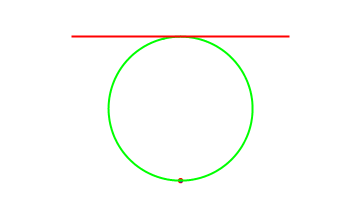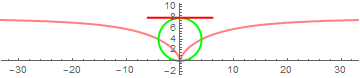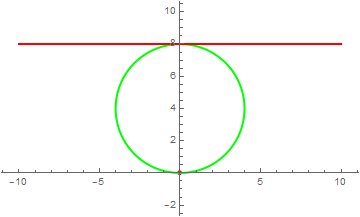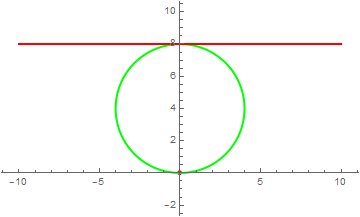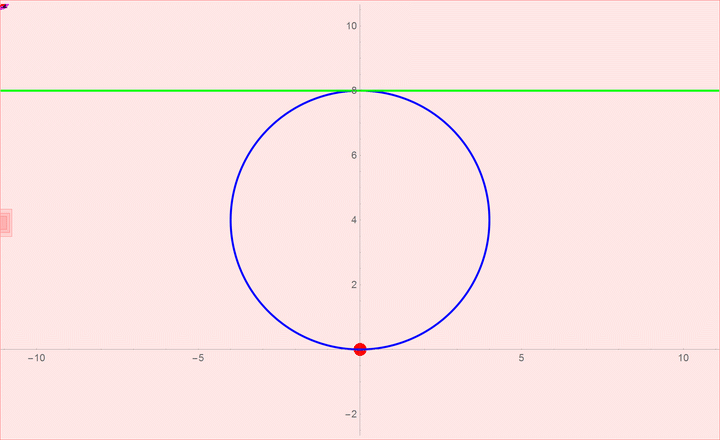a = Table[
Show[{ParametricPlot[{(8/Tan[t]) - 4 Sin[2 t], 4 + 4*Cos[2 t]}, {t,
0.01, t}, PlotStyle -> {Thick, RGBColor[1, 0, 1]},
AxesOrigin -> {0, 0}]},
{Graphics[{Red, Disk[{4*Sin[2 t], 4 - 4*Cos[2 t]}, 0.2]}]},
Graphics[{{RGBColor[1, 0, 1],
Disk[{(8/Tan[t]) - 4 Sin[2 t], 4 + 4*Cos[2 t]}, 0.25]},
Text[Style["M", Black, Italic, FontFamily -> "Times",
22], {(8/Tan[t]) - 4 Sin[2 t] + 0.1, 4 + 4*Cos[2 t] + 0.6}]}],
Graphics[{{Red, Disk[{8/Tan[t], 8}, 0.2]},
Text[Style["O", Black, Italic, FontFamily -> "Times",
22], {0, -0.6}]}],
ListLinePlot[{{(8/Tan[t]) - 4 Sin[2 t], 4 + 4*Cos[2 t]}, {8/Tan[t],
8}}, PlotStyle -> {Thick, Red}],
ListLinePlot[{{0, 0}, {4*Sin[2 t], 4 - 4*Cos[2 t]}},
PlotStyle -> {Thick, Red}],
ListLinePlot[{{4*Sin[2 t],
4 - 4*Cos[2 t]}, {(8/Tan[t]) - 4 Sin[2 t], 4 + 4*Cos[2 t]}},
PlotStyle -> {Thick, RGBColor[0, 1, 0.501961]}],
PolarPlot[8 Sin[x], {x, 0, Pi}, PlotStyle -> {Thick, Blue}],
Plot[8, {x, -12, 12}, PlotStyle -> {Thick, Green}],
PlotRange -> {{-10, 10}, {-2, 10}}], {t, 0, Pi, 0.5/10}]
Export["e:/Program Files/math files/蔓叶线34.gif", a,
ImageSize -> {720, 440}, ImageResolution -> 300]12-03
05-059805
08-23988
05-082091
12-14582
10-27
05-06
01-18